# Invariants and Performance CS 5010 Program Design Paradigms

• Slides: 18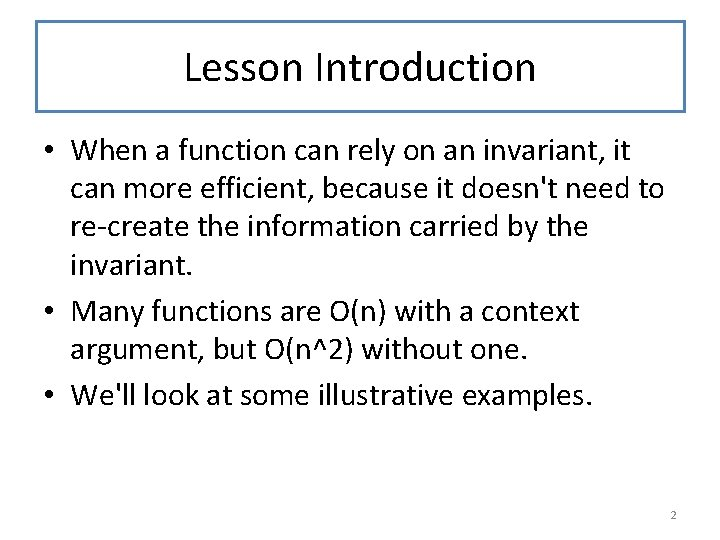Lesson Introduction • When a function can rely on an invariant, it can more efficient, because it doesn't need to re-create the information carried by the invariant. • Many functions are O(n) with a context argument, but O(n^2) without one. • We'll look at some illustrative examples. 2Learning Objectives • At the end of this lesson the student should be able to – show two examples of functions that can be written either with context arguments or without them – explain why the version with context arguments are far more efficient – explain how context arguments and invariants can lead to better designs. 3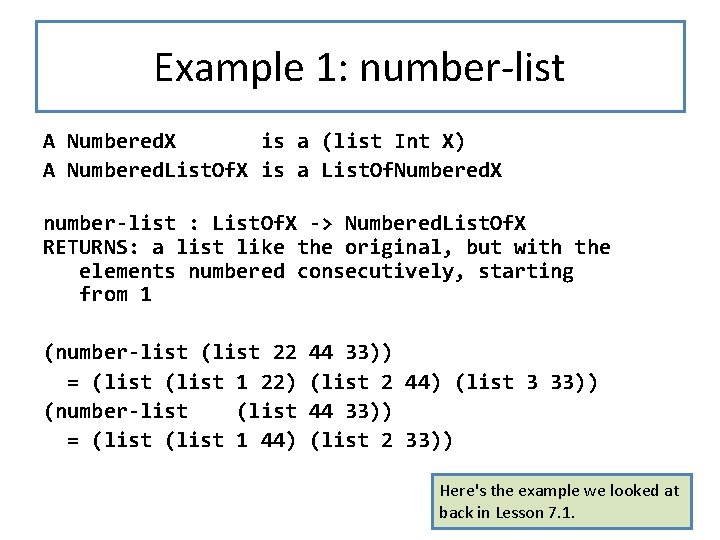Example 1: number-list A Numbered. X is a (list Int X) A Numbered. List. Of. X is a List. Of. Numbered. X number-list : List. Of. X -> Numbered. List. Of. X RETURNS: a list like the original, but with the elements numbered consecutively, starting from 1 (number-list (list 22 = (list 1 22) (number-list (list = (list 1 44) 44 33)) (list 2 44) (list 3 33)) 44 33)) (list 2 33)) Here's the example we looked at back in Lesson 7. 1. 4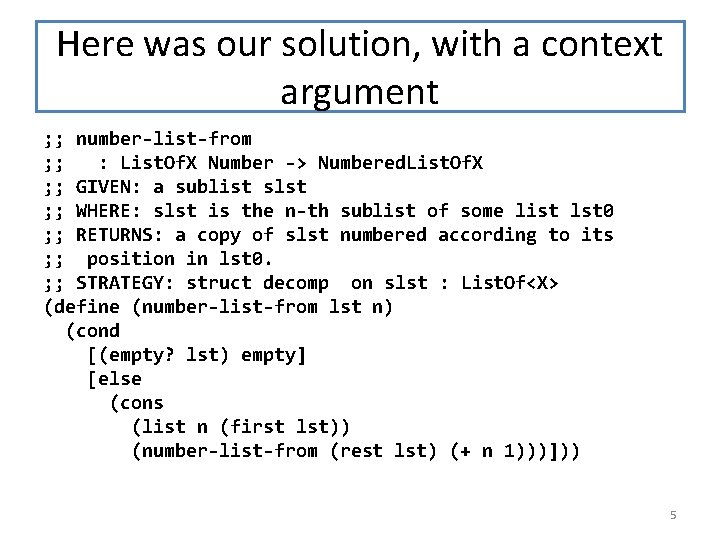Here was our solution, with a context argument ; ; number-list-from ; ; : List. Of. X Number -> Numbered. List. Of. X ; ; GIVEN: a sublist slst ; ; WHERE: slst is the n-th sublist of some list lst 0 ; ; RETURNS: a copy of slst numbered according to its ; ; position in lst 0. ; ; STRATEGY: struct decomp on slst : List. Of<X> (define (number-list-from lst n) (cond [(empty? lst) empty] [else (cons (list n (first lst)) (number-list-from (rest lst) (+ n 1)))])) 5Could we do this directly? (define (number-list lst) (cond [(empty? lst) empty] [else (number-list-combiner (first lst) (number-list (rest lst)))])) What must number-list-combiner do? Let's look at our example. 6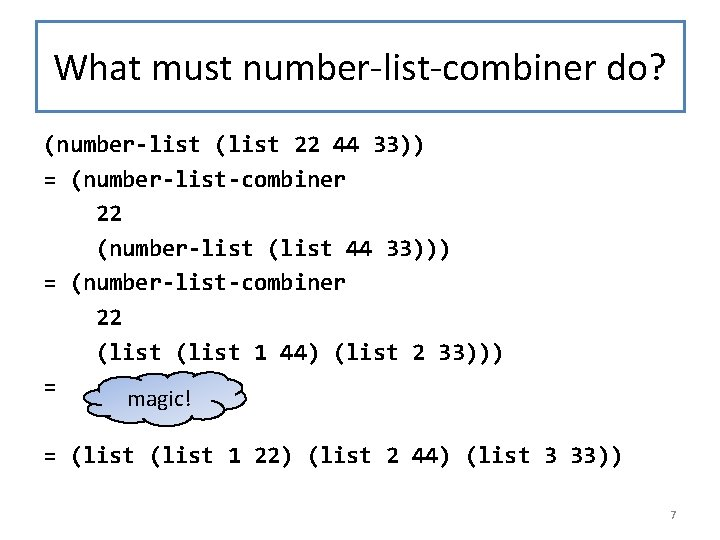What must number-list-combiner do? (number-list (list 22 44 33)) = (number-list-combiner 22 (number-list (list 44 33))) = (number-list-combiner 22 (list 1 44) (list 2 33))) = magic! = (list 1 22) (list 2 44) (list 3 33)) 7What must number-list-combiner do? (number-list-combiner 22 (list 1 44) (list 2 33))) = (list 1 22) (list 2 44) (list 3 33)) I see a map here And a cons here 8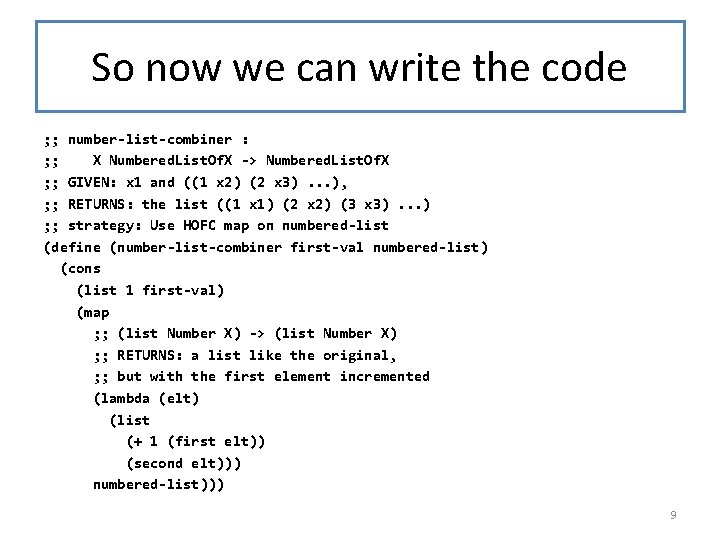So now we can write the code ; ; number-list-combiner : ; ; X Numbered. List. Of. X -> Numbered. List. Of. X ; ; GIVEN: x 1 and ((1 x 2) (2 x 3). . . ), ; ; RETURNS: the list ((1 x 1) (2 x 2) (3 x 3). . . ) ; ; strategy: Use HOFC map on numbered-list (define (number-list-combiner first-val numbered-list) (cons (list 1 first-val) (map ; ; (list Number X) -> (list Number X) ; ; RETURNS: a list like the original, ; ; but with the first element incremented (lambda (elt) (list (+ 1 (first elt)) (second elt))) numbered-list))) 9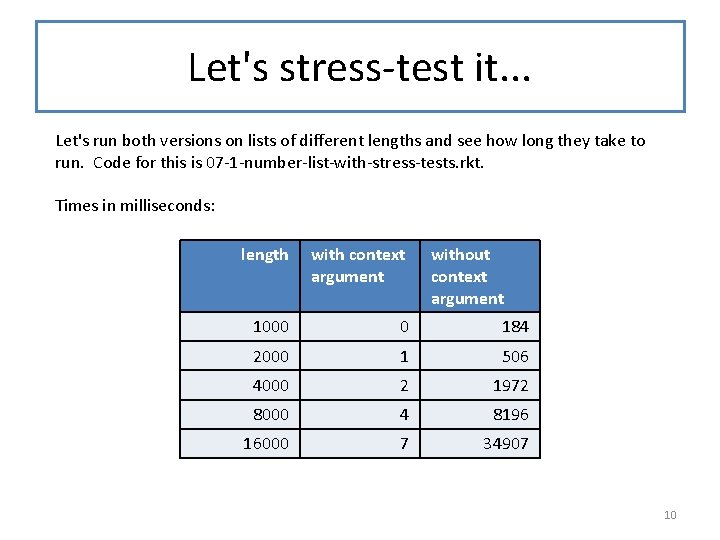Let's stress-test it. . . Let's run both versions on lists of different lengths and see how long they take to run. Code for this is 07 -1 -number-list-with-stress-tests. rkt. Times in milliseconds: length with context argument without context argument 1000 0 184 2000 1 506 4000 2 1972 8000 4 8196 16000 7 34907 10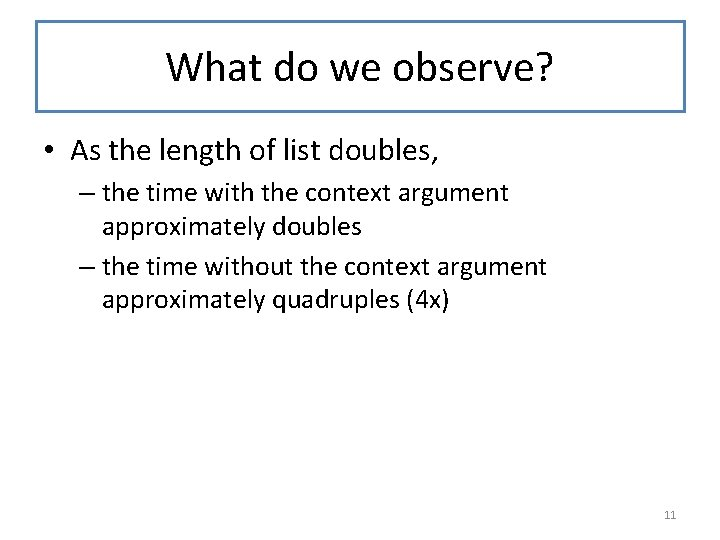What do we observe? • As the length of list doubles, – the time with the context argument approximately doubles – the time without the context argument approximately quadruples (4 x) 11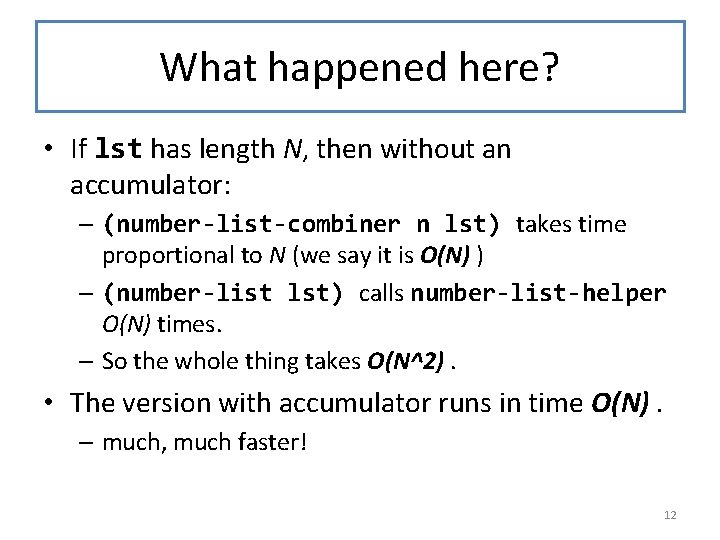What happened here? • If lst has length N, then without an accumulator: – (number-list-combiner n lst) takes time proportional to N (we say it is O(N) ) – (number-list lst) calls number-list-helper O(N) times. – So the whole thing takes O(N^2). • The version with accumulator runs in time O(N). – much, much faster! 12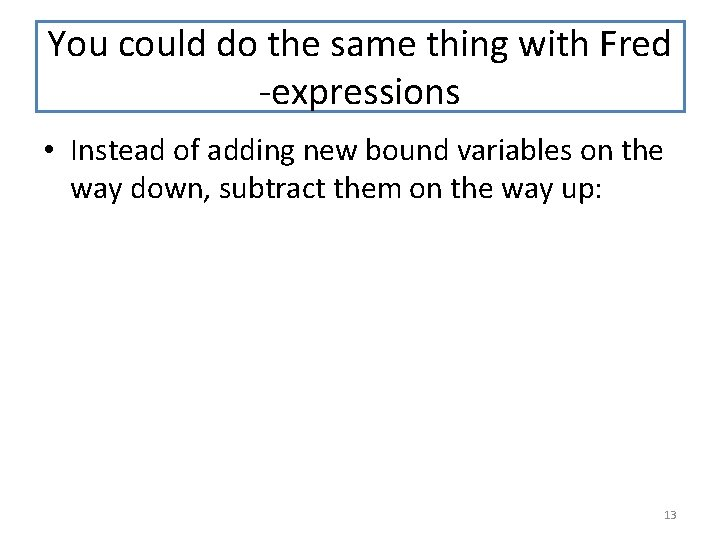You could do the same thing with Fred -expressions • Instead of adding new bound variables on the way down, subtract them on the way up: 13Function Definition ; ; STRATEGY: Use template for Fred. Exp on f (define (free-vars f) (cond [(var? f) (list (var-name f))] [(lam? f) (set-minus (free-vars (lam-body f)) (lam-var f))] [(app? f) (set-union (free-vars (app-fn f)) (free-vars (app-arg f)))])) We can write free-vars as a straightforward structural decomposition, using the set operations from sets. rkt. At each lam, we find all the variables in the body, and then remove the lambda -variable from that set We use set-union, rather than append or something like it, because we are supposed to return a set. 14Fred-expressions Size no context arg with context arg 2559 0 0 81, 919 328 47 655, 358 2528 390 2, 621, 439 10732 1591 We saw similar speedups with the Fred. Exp example. From this evidence, it's clear the version with the context argument runs much faster, but there's not enough data here to see whethere's an asymptotic speedup (eg O(n) vs O(n^2)) 15But performance really isn't the point • The real point of invariants is to document the assumptions that a function makes about the world it lives in. • Many times, those assumptions are things the function cannot check except with great difficulty – e. g. , the order contains no duplicates – e. g. , the inventory is sorted • You want to check these things once, and then the other functions can rely on them. • This also means you have a single point of control for these checks – this leads to a better design 16Summary • You should now be able to – show two examples of functions that can be written either with context arguments or without them – explain why the version with context arguments are far more efficient – explain how context arguments and invariants can lead to better designs. 17